# 深入理解正则化

## 综述

刚开始接触机器学习就提到了正则化，一直没有把里面东西搞清楚。今天决定写一篇关于正则化的文章将里面的问题讲讲清楚。

• 从多种角度认识正则化

• 正则化的作用

• 正则化作用补充

• 正则化参数选择

## 从多种角度认识正则化

##### 1、贝叶斯角度的正则化

LR正则化对应数据先验的分布。比如L1（LASSO）先验对应高斯分布，L2（Ridge）对应拉普拉斯分布。现在通过一个案例说明LR正则化代表对模型参数引入先验分布，来看线性回归：$y=Wx+ϵ$$y=Wx+\epsilon$,之前线性回归已经了解到$y-Wx=ϵ$$y-Wx=\epsilon$对于$ϵ$$\epsilon$服从高斯分布因此：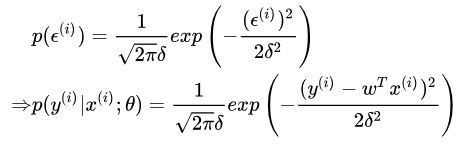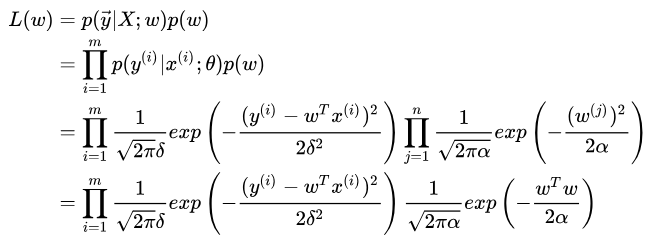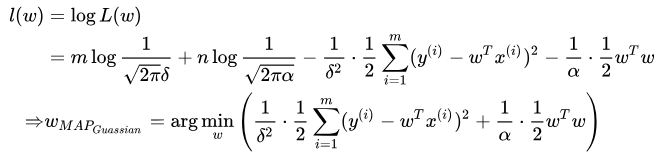上文说完了L2接下来再来看一下L1，首先看一下拉普拉斯分布是个什么鬼？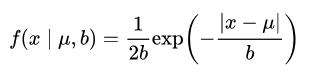对应的函数图像为：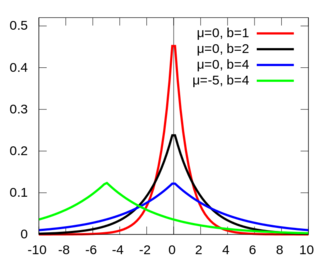观察一下发现与正态分布间的图像很像，有兴趣的可以去查一下与正态分布的关系。我们重复L2中的推导很容易得到：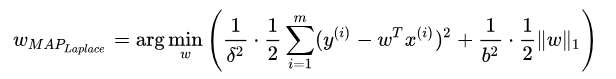观察表达式的右侧得到了L1的正则化项。我们知道贝叶斯模型中可以有效的缓解过拟合问题，通过前面的分析发现，其实贝叶斯学派中对也是有过拟合处理的模块的。贝叶斯的似然函数相当于损失函数，先验分布相当于正则化项。想深入了解的同学可以查看这篇文章——Lazy Sparse Stochastic Gradient Descent for Regularized Mutlinomial Logistic Regression

##### 2、结构风险最小化

这里的想法很简单，就是为了缓解风险加上一个正则化项,通过添加一个正则化项降低参数的大小，让各个参数起的作用小点那么整体的模型趋于简单。但是通过这个角度我们可以很好的解释不同正则化项起的作用。

## 正则化的多种作用

我们经常使用的正则化参数有L0，L1，L2三种，其中L0与L1作用相似，但是L1更易于优化所以我们一般直接使用L1，本文也就常用的两种正则化项进行说明。

##### 1. L1——（Lasso regularization）的作用

L1范数是指向量中各个元素绝对值之和，也有个美称叫“稀疏规则算子”（Lasso regularization）。为什么L1范数会使权值稀疏？任何的规则化算子，如果他在${w}_{i}=0$$w_i=0$的地方不可微，并且可以分解为一个“求和”的形式，那么这个规则化算子就可以实现稀疏。这说是这么说，$w$$w$的L1范数是绝对值，$|w|$$|w|$在w=0处是不可微，但这还是不够直观。通过一张大家都见过但是没见过有人讲清楚的图的说明一下。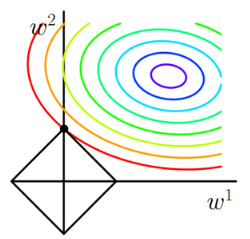在说明这张图之前我们先来分解一下原来的似然函数，我们可以把正则化看做是损失函数的一个约束。这样逆推拉格朗日乘子法得到如下表达式：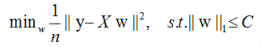这样把又表述一个带约束的线性规划问题。但是问题的目的一定要清晰就是通过调整参数$w$$w$的值来得到最小化的优化目标值。以二维问题为例，将两个方程分别画在一个以${w}_{1},{w}_{2}$$w_1,w_2$为坐标轴的平面内，其中黑色方框表示约束条件的形状。右上角一圈一圈的是损失函数的图像，损失函数是凸函数所以越往外层是函数值增大的方向，图中的方向选择等高线的法向量方向
现在的问题就转化成了求解两个图像的交点问题，因为参数值没有确定我们仅能构建出一个大致的形状，通过求解出交点后才可以确定最终图像的精确模样。那么为什么最有解一定在约束图像的顶点处呢？(在凸优化课程中有对于这一命题的详细论证，感兴趣的可以查看Boyd的书)我们看一个案例：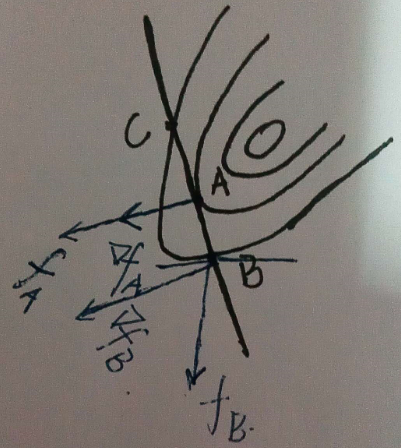观察上图，黑色的直线表示约束方程，方程与等高线相切于A点${f}_{A}$$f_A$表示A点的法向量方向也就是函数值增大的方向，$\vee {f}_{A}$$\vee f_A$表示A点的梯度方向，梯度方向表示损失函数在这一点坡度最大的方向。观察图中的B点，${f}_{B}$$f_B$表示B点的法向量方向，$\vee {f}_{B}$$\vee f_B$表示该点的梯度方向。B点所处的等高线要高于A点，也就是A点的得到的损失函数值要小于B点。我们的目标就是要最小化损失函数，所以A点要比B好的多。但是A点是不是最终的解，这里先给出答案——是的，是最好的点。观察A、B两点的等高线法向量方向和梯度方向有什么不同呢？A点法线和梯度共线了！B点成为一个夹角了！也就是说当法向量与梯度方向共线了这个点就是我们所要求解的点。也就是说当法向量与梯度方向存在夹角时就还有优化的空间，上图中如果在A，B两点所在的等高线间再加入一条等高线并在等高线上再选一点D发现，D的法向量与梯度的夹角又变小了，我们不断的优化一直到法向量与梯度的夹角为0时获得了最好的结果。
那好现在重新回头来看这个问题，现在再添加一条登高线（勿喷）：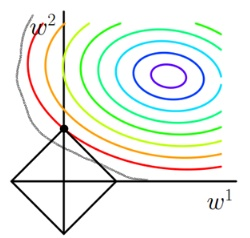新添加的等高线，与约束区域有多个交点，用上文分析的方法发现只有图中的顶点时得到函数值是最小的。
终于大体说明白了为啥图中交点最小的问题。现在来看问啥L1正则可以得到稀疏解呢？观察图中最小值点的坐标$（0,{w}_{2}）$$（0,w_2）$发现${w}_{1}$$w_1$为0，也就是得到的最优值的点只有${w}_{2}$$w_2$起作用，设想在高维的情况下，会有更多的参数等于零。这样就是L1得到稀疏解的来源！换种说法就是L1范数可以自己选择重要的特征参与运算。

##### 1. L2的作用

说清楚L1的作用再说L2就轻松多了。还是直接上图：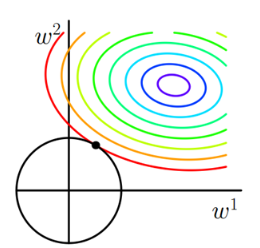观察约束是个圆形，这个时候最优解取到坐标轴上的可能性是比较小的。所以L2正则化最大主要目的是使得各个参数趋向于0，这样会得到一条更加光滑的函数。因为一般认为参数值小的模型比较简单，能适应不同的数据集，也在一定程度上避免了过拟合现象。可以设想一下对于一个线性回归方程，若参数很大，那么只要数据偏移一点点，就会对结果造成很大的影响；但如果参数足够小，数据偏移得多一点也不会对结果造成什么影响，专业一点的说法是『抗扰动能力强』。

接下来通过公式再来看一下这个问题，最小化损失是通过梯度下降一步步求解的，我们先看L2的梯度更新公式：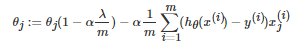没有L2正则项时，参数更新公式没有公式右侧的第一项。加入正则化后，发现参数先乘上一个小于1的数然后再减去后面的一坨。这样参数下降的就更多了。而只有L1正则时，

${\theta }_{j}:={\theta }_{j}-\alpha \frac{1}{m}\sum \left(h-y\right){x}_{j}+\frac{\lambda }{m}$

## 正则化参数选择

通常越大的λ可以让代价函数在参数为0时取到最小值。下面是一个简单的例子，这个例子来自Quora上的问答。为了方便叙述，一些符号跟这篇帖子的符号保持一致。
假设有如下带L1正则化项的代价函数：

$F\left(x\right)=f\left(x\right)+\lambda {‖x‖}_{1}$

其中x是要估计的参数，相当于上文中提到的$w$$w$以及$\theta$$θ$. 注意到$L1$$L1$正则化在某些位置是不可导的，当$\lambda$$λ$足够大时可以使得$F\left(x\right)在x=0$$F(x)在x=0$时取到最小值。如下图：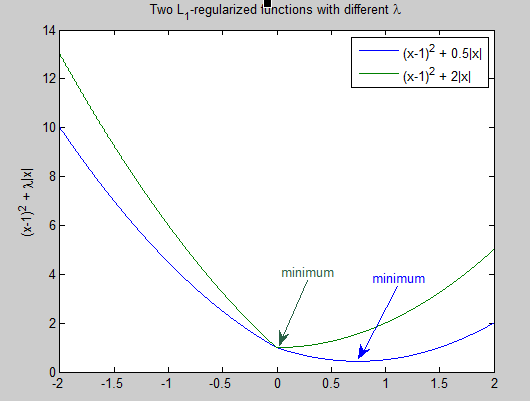分别取$\lambda =0.5和\lambda =2$$λ=0.5和λ=2$，可以看到越大的$\lambda$$λ$越容易使$F\left(x\right)在x=0$$F(x)在x=0$时取到最小值。

L2正则化参数;参考图2，λ越大，L2圆的半径越小，最后求得代价函数最值时各参数也会变得很小。